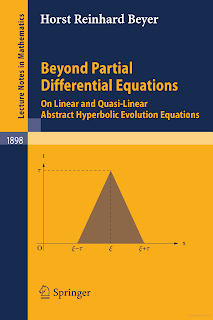DISCRETE MATHEMATICS

# Beyond Partial Differential Equations on Linear and Quasi Linear Abstract Hyperbolic Evolution Equations Free DownloadSemigroup Theory uses abstract methods of Operator Theory to treat initial boundary value problems for linear and nonlinear equations that describe the evolution of a system. Due to the generality of its methods, the class of systems that can be treated in this way exceeds by far those described by equations containing only local operators induced by partial derivatives, i.e., PDEs. In particular, that class includes the systems of Quantum Theory. Another important application of semi group methods is in quantization. Simple examples are given by the cases of free in Minkowski spacetime like Klein-Gordon the Diracted and the Maxwell whose equations are given by systems of linear PDEs. The second quantization of such a replaces the equation by a Schrodinger equation whose Hamilton operator is given by the second quantization of a non-local function of a self-adjoint linear operator. That operator generates the semigroup given by the time-development of the solutions of the equation corresponding to arbitrary initial data as a function of time.